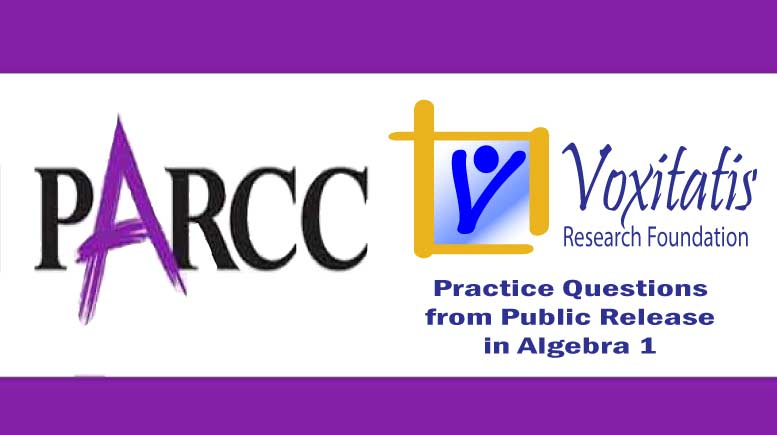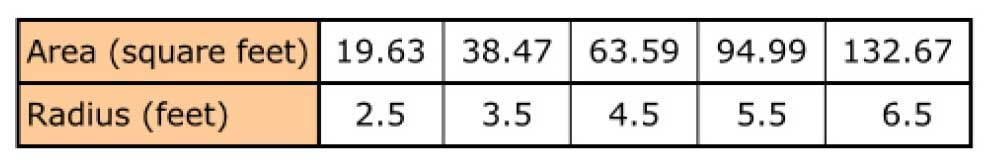Friday, September 17, 2021# Algebra 1 PARCC question: table rate of change

-#### The following fill-in-the-blank question, explained here in hopes of helping algebra students in Maryland and Illinois prepare for the PARCC test near the end of this school year, appears on the released version of PARCC’s Spring 2015 test in algebra 1, here:

Carrie wants to find out how the area of a circle will change as the radius increases in length. Carrie makes a table.What is the average rate of change in the area as the radius changes from 2.5 to 5.5 feet?

_____ square feet per foot

Correct answer: 25.12 square feet per foot

PARCC evidence statement(s) tested: F-IF.6-1a:

Calculate and interpret the average rate of change of a function (presented symbolically or as a table) over a specified interval with functions limited to linear, exponential (with domains in the integers), and quadratic functions.

Tasks have a real-world context. Tasks must include the interpret part of the evidence statement.

The evidence statement above references Math Practice 1, Math Practice 4, Math Practice 5, and Math Practice 7.

The question tests students’ partial understanding of the eighth-grade Common Core math standard 8.F.B.4, which states that they should be able to “model a linear relationship between two quantities” and “determine the rate of change and initial value of the function from a description of a relationship or from two (x, y) values, including reading these from a table or from a graph. Interpret the rate of change and initial value of a linear function in terms of the situation it models, and in terms of its graph or a table of values.” The fact that this isn’t a linear relationship is irrelevant (see analysis below).

Solution strategy (there are others)

Determine from the table how much the area changed per unit of radius.

Since the “per” unit is the feet of the radius, how many units are we asked to consider? 5.5 feet – 2.5 feet = 3 feet. So, 3 units of radius.

Over that change, the area of the circle increased from 19.63 square feet to 94.99 square feet, or 75.36 square feet.

The units in the table are the same units in which we’re asked to express the answer, so no conversion is required. To get the average rate of change, we can simply divide the change in area by the change in radius:$\frac{94.99 - 19.63}{5.5 - 2.5} = \frac{75.36}{3} = 25.12$

## Resources for further study

Purple Math, developed by Elizabeth Stapel, a math teacher from the St Louis area, has a very nice description of what the slope and y intercept almost always mean in the context of a word problem like the one posed by this PARCC question. The page can be found here.

The Khan Academy, developed by Sal Khan, an engineer who has created a library of thousands of video lessons, has one that describes how Jordan can’t help but adopt more cats, which she does at a constant rate in the word problem he solves. What do the slope and y intercept mean in this context? He explains here.

Chapter 2, Section 2.2 of the book Algebra 2, Illinois edition by Ron Larson et al deals with slope and the rate of change. The authors use a Sequoia tree with a trunk that has a diameter of 137 inches in 1965 and 141 inches in 2005. The average rate of change, R, in inches per year, is given by the formula$R = \frac{d}{y}$

where d is the change in diameter, and y is the number of years. To find the average rate of change, also known as the growth rate of the tree, in inches per year, we can use$R = \frac{(141 - 137)}{(2005 - 1965)} = \frac{4}{40} = 0.1$

The trunk grows, on average, 0.1 inches in diameter every year.

Complete reference: Ron Larson, Laurie Boswell, Timothy D Kanold, Lee Stiff. Algebra 2, Illinois edition. Evanston, Ill.: McDougal Littell, a division of Houghton Mifflin Company, 2008. The book is used in several algebra classes taught in Illinois high schools.

## Analysis of this question and online accessibility

The question measures knowledge of the Common Core standard it purports to measure and tests students’ ability to estimate the rate of change in a linear relationship given a table of (x,y) values. It is considered to have a low cognitive demand.

However, the question is not aligned to the PARCC evidence statement in two important ways, as follows:

(1) The PARCC evidence statement requires a real-world context. The context of the question, Carrie’s desire to determine how much the area of a circle changes per unit radius, is vapid, void and irrelevant to any real-world situation. A search of the database of jobs on the website for the US Bureau of Labor Statistics fails to find any job where such a skill would be required.

(2) The PARCC evidence statement requires that the “interpret” part of the evidence statement be included in the task, in addition to the calculation of the average rate of change. There is no interpretation of the rate of change involved in the problem.

Note that since students never have to recognize this as a quadratic relationship between the two quantities, the question does not align to the Common Core high school algebra standard HSF.IF.B.4: “For a function that models a relationship between two quantities, interpret key features of graphs and tables in terms of the quantities, and sketch graphs showing key features given a verbal description of the relationship. Key features include: intercepts; intervals where the function is increasing, decreasing, positive, or negative; relative maximums and minimums; symmetries; end behavior; and periodicity.” Several important parts of this standard are not assessed here and may be assessed on other test questions.

The question can be tested online and should yield results that are as valid and reliable as those obtained on paper, although the fill-in-the-blank format requires online testing so the student response can be scored automatically.

No special accommodation challenges can be identified with this question, so the question is considered fair.

## Challenge

The formula for the area (A) of a circle, given the radius (r), is$A = \pi r^2$

What is the connection of this formula to the table above?

## Purpose of this series of posts

Voxitatis is developing blog posts that address every algebra 1 question released to the public by the Partnership for Assessment of Readiness for College and Careers, or PARCC, in order to help students prepare to take the test this spring.

Our total release will run from February 27 through March 15, with one or two questions discussed per day. Then we’ll move to geometry at the end of March, algebra 2 during the first half of April, and eighth grade during the last half of April.Paul Katulahttps://news.schoolsdo.org
Paul Katula is the executive editor of the Voxitatis Research Foundation, which publishes this blog. For more information, see the About page.

### Study finds increasing suicide rates among Black girls

0
Why are Black adolescent girls committing suicide at increasingly higher rates in the US? Could it be an effect of racism and prejudice?